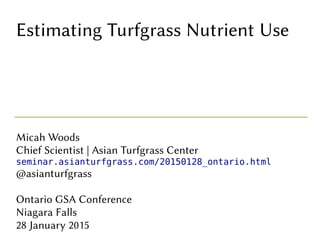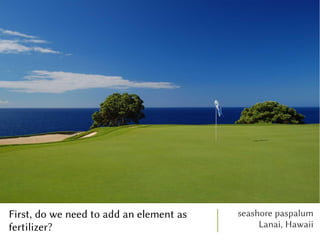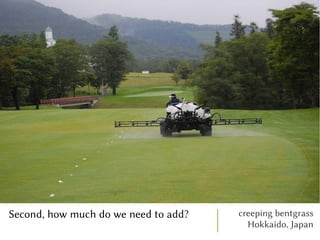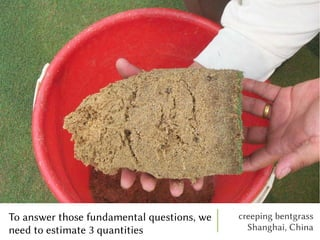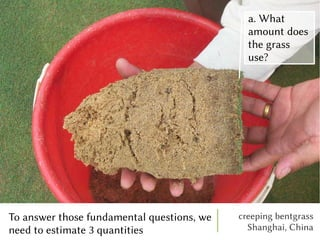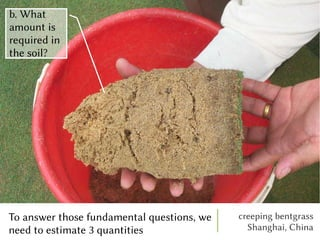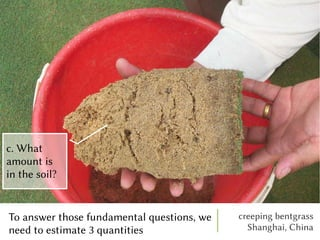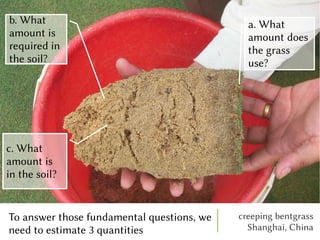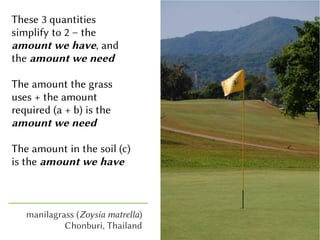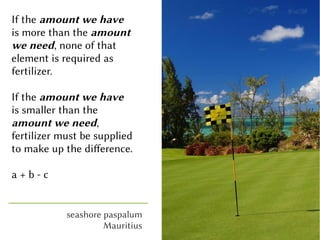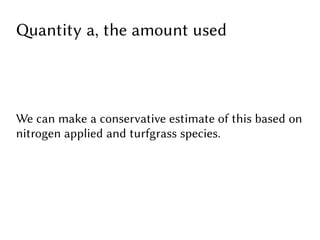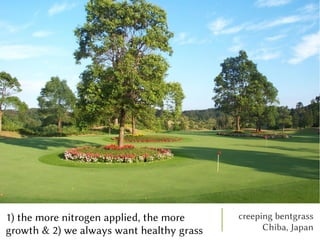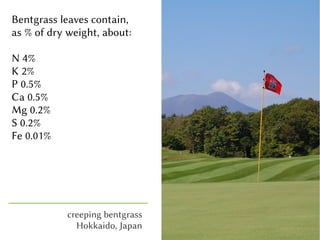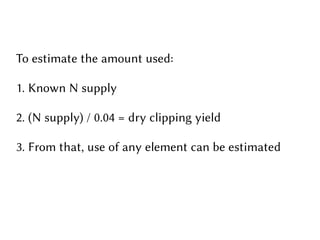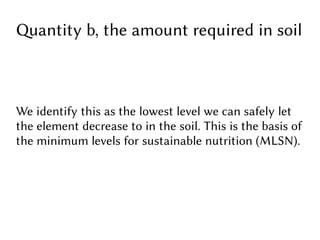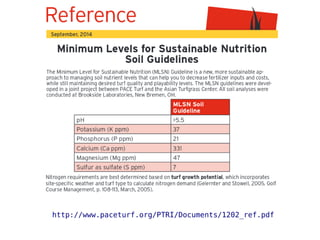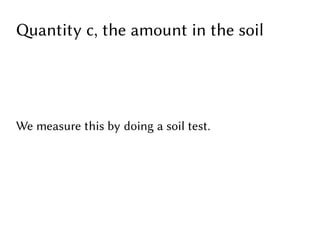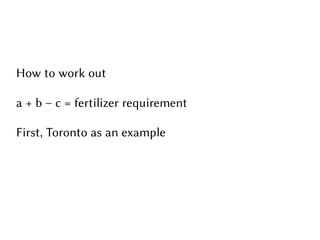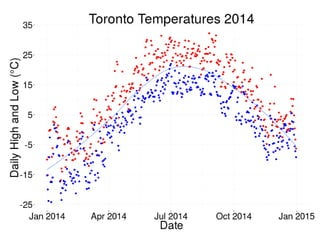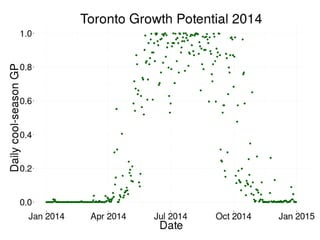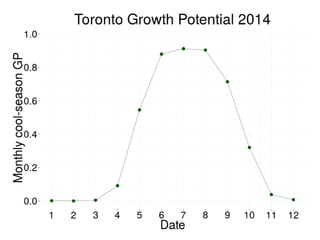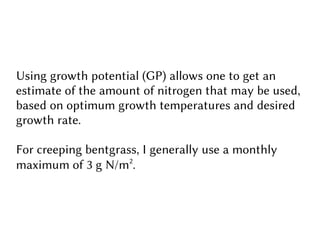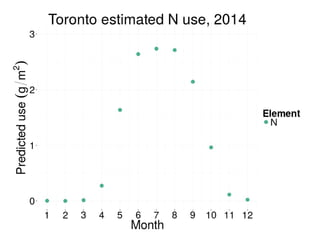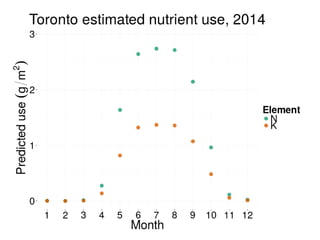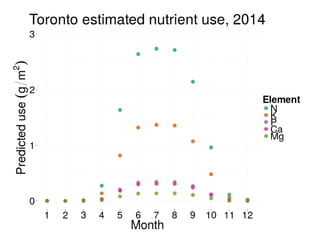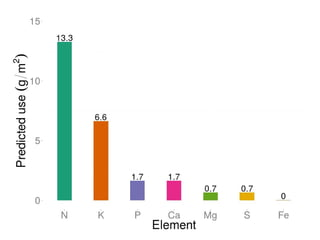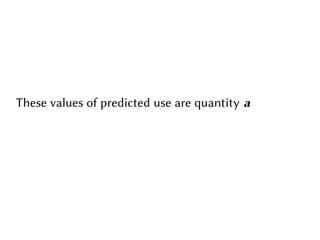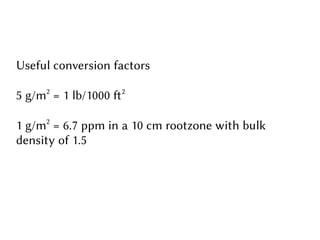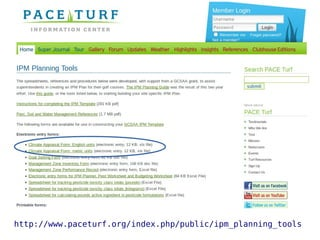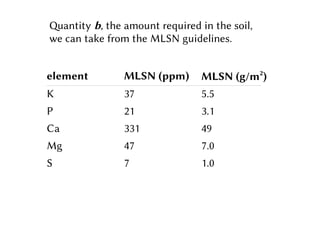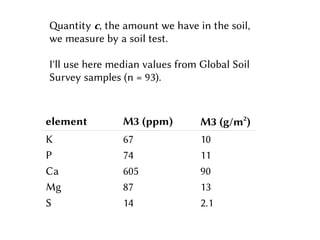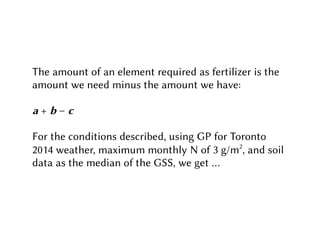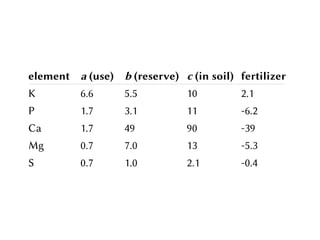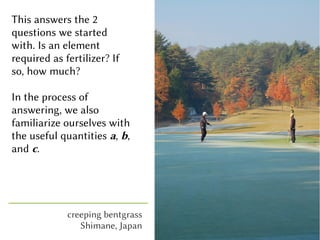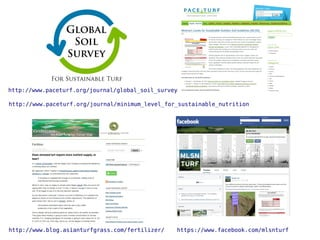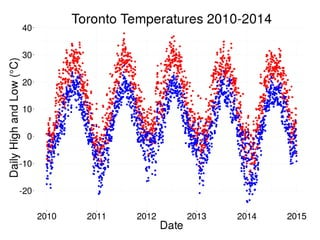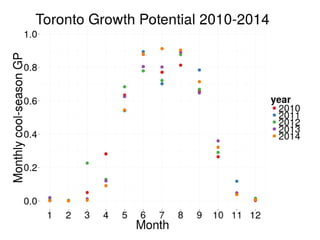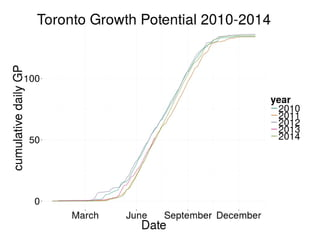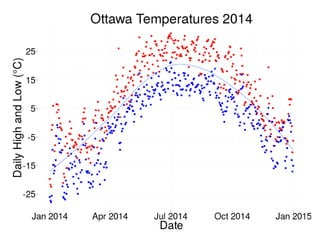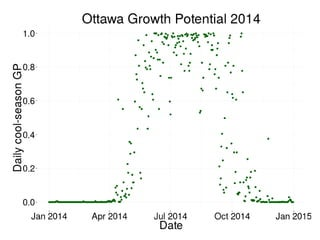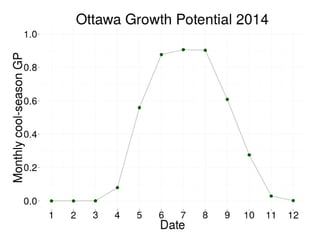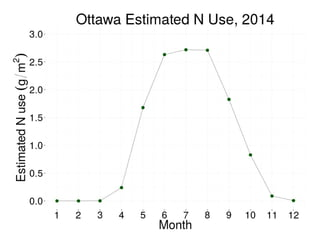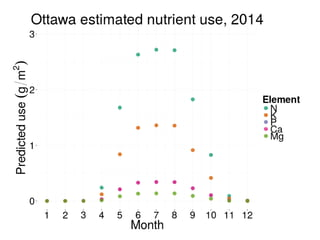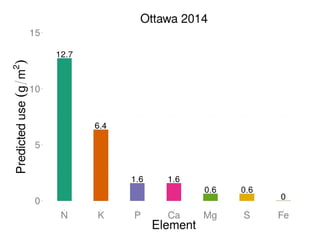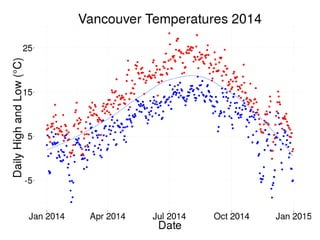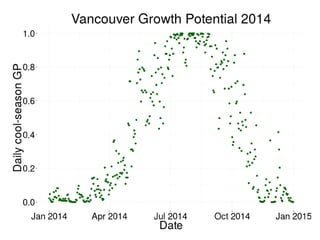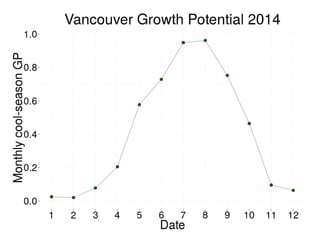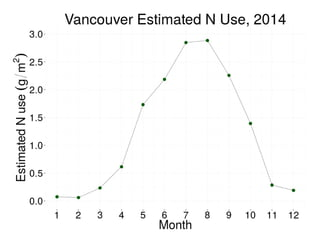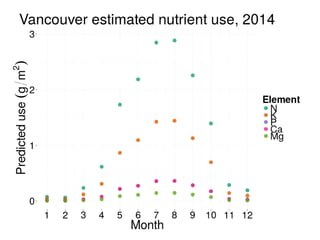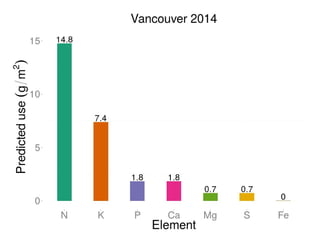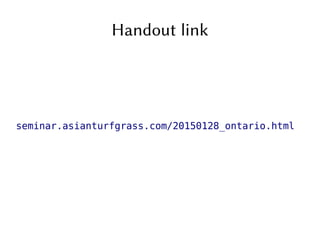1 of 51

### Estimating Turfgrass Nutrient Use

1. Estimating Turfgrass Nutrient Use Micah Woods Chief Scientist | Asian Turfgrass Center seminar.asianturfgrass.com/20150128_ontario.html @asianturfgrass Ontario GSA Conference Niagara Falls 28 January 2015
2. First, do we need to add an element as fertilizer? seashore paspalum Lanai, Hawaii
3. Second, how much do we need to add? creeping bentgrass Hokkaido, Japan
4. To answer those fundamental questions, we need to estimate 3 quantities creeping bentgrass Shanghai, China
5. To answer those fundamental questions, we need to estimate 3 quantities creeping bentgrass Shanghai, China
6. To answer those fundamental questions, we need to estimate 3 quantities creeping bentgrass Shanghai, China
7. To answer those fundamental questions, we need to estimate 3 quantities creeping bentgrass Shanghai, China
8. To answer those fundamental questions, we need to estimate 3 quantities creeping bentgrass Shanghai, China
9. These 3 quantities simplify to 2 – the amount we have, and the amount we need The amount the grass uses + the amount required (a + b) is the amount we need The amount in the soil (c) is the amount we have manilagrass (Zoysia matrella) Chonburi, Thailand
10. If the amount we have is more than the amount we need, none of that element is required as fertilizer. If the amount we have is smaller than the amount we need, fertilizer must be supplied to make up the difference. a + b - c seashore paspalum Mauritius
11. Quantity a, the amount used We can make a conservative estimate of this based on nitrogen applied and turfgrass species.
12. 1) the more nitrogen applied, the more growth & 2) we always want healthy grass creeping bentgrass Chiba, Japan
13. Bentgrass leaves contain, as % of dry weight, about: N 4% K 2% P 0.5% Ca 0.5% Mg 0.2% S 0.2% Fe 0.01% creeping bentgrass Hokkaido, Japan
14. To estimate the amount used: 1. Known N supply 2. (N supply) / 0.04 = dry clipping yield 3. From that, use of any element can be estimated
15. Quantity b, the amount required in soil We identify this as the lowest level we can safely let the element decrease to in the soil. This is the basis of the minimum levels for sustainable nutrition (MLSN).
16. http://www.paceturf.org/PTRI/Documents/1202_ref.pdf
17. Quantity c, the amount in the soil We measure this by doing a soil test.
18. How to work out a + b – c = fertilizer requirement First, Toronto as an example
19. Using growth potential (GP) allows one to get an estimate of the amount of nitrogen that may be used, based on optimum growth temperatures and desired growth rate. For creeping bentgrass, I generally use a monthly maximum of 3 g N/m2 .
20. These values of predicted use are quantity a
21. Useful conversion factors 5 g/m2 = 1 lb/1000 f2 1 g/m2 = 6.7 ppm in a 10 cm rootzone with bulk density of 1.5
22. http://www.paceturf.org/index.php/public/ipm_planning_tools
23. element MLSN (ppm) MLSN (g/m2 ) K 37 5.5 P 21 3.1 Ca 331 49 Mg 47 7.0 S 7 1.0 Quantity b, the amount required in the soil, we can take from the MLSN guidelines.
24. element M3 (ppm) M3 (g/m2 ) K 67 10 P 74 11 Ca 605 90 Mg 87 13 S 14 2.1 Quantity c, the amount we have in the soil, we measure by a soil test. I'll use here median values from Global Soil Survey samples (n = 93).
25. The amount of an element required as fertilizer is the amount we need minus the amount we have: a + b – c For the conditions described, using GP for Toronto 2014 weather, maximum monthly N of 3 g/m2 , and soil data as the median of the GSS, we get .
26. element a (use) b (reserve) c (in soil) fertilizer K 6.6 5.5 10 2.1 P 1.7 3.1 11 -6.2 Ca 1.7 49 90 -39 Mg 0.7 7.0 13 -5.3 S 0.7 1.0 2.1 -0.4
27. This answers the 2 questions we started with. Is an element required as fertilizer? If so, how much? In the process of answering, we also familiarize ourselves with the useful quantities a, b, and c. creeping bentgrass Shimane, Japan